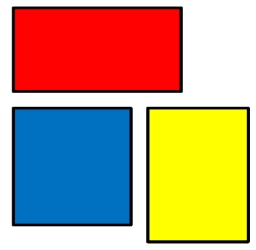# Rectangle 83

Rectangle land has a dimension of 8 km times 10 9/10 km. What is the area?

Write your answer as a fraction or as a whole or mixed number.

A =  87 15 = 436/5 km2

### Step-by-step explanation:Did you find an error or inaccuracy? Feel free to write us. Thank you!

Tips for related online calculators
Need help calculating sum, simplifying, or multiplying fractions? Try our fraction calculator.
Need help with mixed numbers? Try our mixed-number calculator.

#### Grade of the word problem:

We encourage you to watch this tutorial video on this math problem: